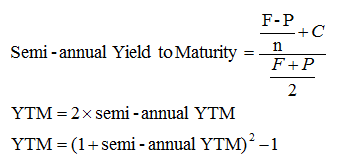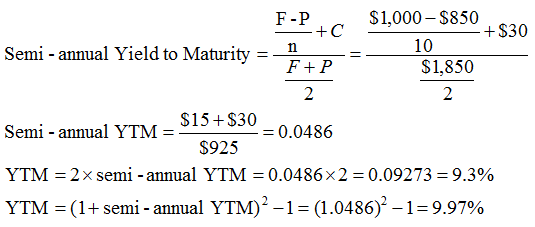# Yield to Maturity (YTM) Approximation Formula

Calculating yield to maturity can sometimes be a tedious process because we need to do trial and error to arrive at the exact yield.

In this article we provide an approximation formula to calculate YTM that you can use for quick calculation. The following formula assumes semi-annual coupon payments.Where:

• F = Face Value = Par Value (Usually $1,000) • P = Bond Price • C = the semi-annual coupon interest • N = number of semi-annual periods left to maturity Let’s take an example to understand how to use the formula. Let us find the yield-to-maturity of a 5 year 6% coupon bond that is currently priced at$850. The calculation of YTM is shown below:Note that the actual YTM in this example is 9.87%. However, our approximation is good enough for exams or for quick comparisons.

Get smart about tech at work.

As a non-technical professional, learn how software works with simple explanations of tech concepts. Learn more...

# Data Science for Finance Bundle: 43% OFF

Get our Data Science for Finance Bundle for just $29$51.